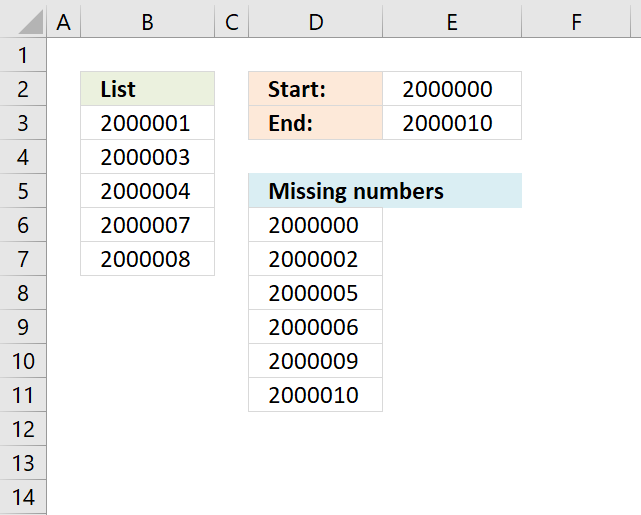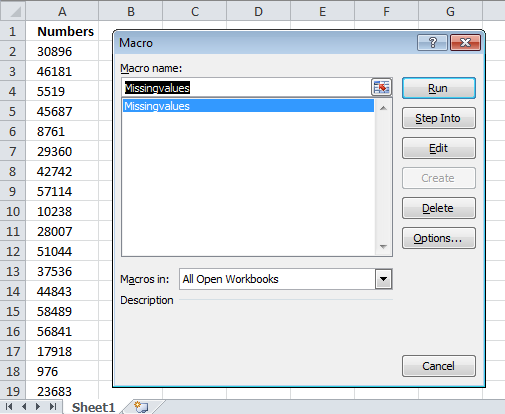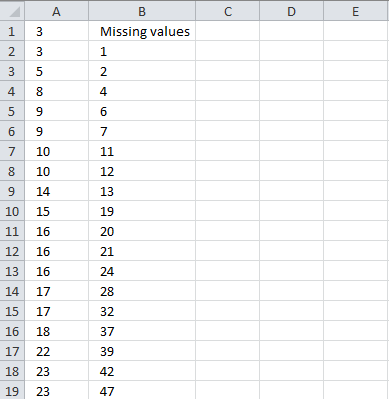Author: Oscar Cronquist Article last updated on January 03, 2019The image above shows an array formula in cell D6 that extracts missing numbers i cell range B3:B7, the lower boundary is specified in cell E2 and the upper boundary is in cell E3.  The ROW function has a limit of 1 048 576 so the number of values between the lower and upper boundary can't be more than 1 048 576.

If this limit won't work for you then later in this article you will find a macro that doesn't have this limit.

Array Formula in D6

=SMALL(IF(ISERROR(MATCH(\$E\$2+ROW(OFFSET(\$B\$2, 0,0, \$E\$3-\$E\$2+1))-2, \$B\$3:\$B\$7,0)), \$E\$2+ROW(OFFSET(\$B\$2,0, 0, \$E\$3-\$E\$2+1))-2), ROW(A1))

How to create an array formula

1. Select cell D6
2. Copy / Paste array formula
3. Press and hold Ctrl + Shift
4. Press Enter
5. Release all keys

How to copy array formula

1. Copy cell D6
2. Paste to cells below as far as needed.

### Explaining formula in cell B5

#### Step 1 - Build cell reference

The OFFSET function lets you create a cell reference with the same size as there are numbers between 2000000 and 2000010.

OFFSET(\$B\$2,0,0,\$E\$3-\$E\$2+1)

becomes

OFFSET(\$B\$2,0,0,2000010-2000000+1)

becomes

OFFSET(\$B\$2,0,0,11)

and returns \$B\$2:\$B\$12

#### Step 2 - Convert cell reference to row numbers

The ROW function creates an array of row numbers based on the cell reference.

ROW(OFFSET(\$B\$2,0,0,\$E\$3-\$E\$2+1))-2

becomes

ROW(\$B\$2:\$B\$12)-2

becomes

{2;3;4;5;6;7;8;9;10;11;12}-2

and returns

{0;1;2;3;4;5;6;7;8;9;10}

#### Step 3 - Add start number to array

\$E\$2+ROW(OFFSET(\$B\$2,0,0,\$E\$3-\$E\$2+1))-2

becomes

\$E\$2+{0;1;2;3;4;5;6;7;8;9;10}

becomes

2000000+{0;1;2;3;4;5;6;7;8;9;10}

and returns

{2000000;2000001;2000002;2000003;2000004;2000005;2000006;2000007;2000008;2000009;2000010}

This is the list we need to figure out which values are missing.

#### Step 4 - Which values exist?

The MATCH function finds the relative position of each number in the array in cell range \$B\$3:\$B\$7, it returns an error if not found.

MATCH(\$E\$2+ROW(OFFSET(\$B\$2,0,0,\$E\$3-\$E\$2+1))-2,\$B\$3:\$B\$7,0)

becomes

MATCH({2000000;2000001;2000002;2000003;2000004;2000005;2000006;2000007;2000008;2000009;2000010},\$B\$3:\$B\$7,0)

becomes

MATCH({2000000;2000001;2000002;2000003;2000004;2000005;2000006;2000007;2000008;2000009;2000010},{2000001;2000003;2000004;2000007;2000008},0)

and returns

{#N/A;1;#N/A;2;3;#N/A;#N/A;4;5;#N/A;#N/A}

#### Step 5 - Identify errors

The ISERROR function returns TRUE if value is an error and FALSE if not.

ISERROR(MATCH(\$E\$2+ROW(OFFSET(\$B\$2,0,0,\$E\$3-\$E\$2+1))-2,\$B\$3:\$B\$7,0))

becomes

ISERROR({#N/A;1;#N/A;2;3;#N/A;#N/A;4;5;#N/A;#N/A})

and returns

{TRUE;FALSE; TRUE;FALSE; FALSE;TRUE; TRUE;FALSE; FALSE;TRUE; TRUE}.

#### Step 6 - Replace errors with numbers

The IF function converts TRUE to corresponding number.

IF(ISERROR(MATCH(\$E\$2+ROW(OFFSET(\$B\$2,0,0,\$E\$3-\$E\$2+1))-2,\$B\$3:\$B\$7,0)),\$E\$2+ROW(OFFSET(\$B\$2,0,0,\$E\$3-\$E\$2+1))-2)

becomes

IF({TRUE;FALSE; TRUE;FALSE; FALSE;TRUE; TRUE;FALSE; FALSE;TRUE; TRUE},\$E\$2+ROW(OFFSET(\$B\$2,0,0,\$E\$3-\$E\$2+1))-2)

becomes

IF({TRUE;FALSE; TRUE;FALSE; FALSE;TRUE; TRUE;FALSE; FALSE;TRUE; TRUE},{2000000;2000001;2000002;2000003;2000004;2000005;2000006;2000007;2000008;2000009;2000010})

and returns

{2000000; FALSE; 2000002; FALSE; FALSE; 2000005; 2000006; FALSE; FALSE; 2000009; 2000010}

#### Step 7 - Extract k-th smallest number

The SMALL function extracts the k-th smallest number, this makes the formula return a value in a cell each.

SMALL(IF(ISERROR(MATCH(\$E\$2+ROW(OFFSET(\$B\$2,0,0,\$E\$3-\$E\$2+1))-2,\$B\$3:\$B\$7,0)),\$E\$2+ROW(OFFSET(\$B\$2,0,0,\$E\$3-\$E\$2+1))-2),ROW(A1))

becomes

SMALL({2000000; FALSE; 2000002; FALSE; FALSE; 2000005; 2000006; FALSE; FALSE; 2000009; 2000010},ROW(A1))

becomes

SMALL({2000000; FALSE; 2000002; FALSE; FALSE; 2000005; 2000006; FALSE; FALSE; 2000009; 2000010},1)

and returns 2000000 in cell D6.

missing-values-in-a-column-1.xlsx

### Missing numbers (vba)

The macro demonstrated here let´s you select a cell range (values must be in a single column), start and end number.A new sheet is created, values are sorted in the first column. The second column (B) contains all missing values.### VBA

```Sub Missingvalues()
Dim rng As Range
Dim rng1 As Range
Dim StartV As Double, EndV As Double, i As Double, j As Single
Dim k() As Double
Dim WS As Worksheet
ReDim k(0)
On Error Resume Next
Set rng = Application.InputBox(Prompt:="Select a range:", _
Title:="Extract missing values", _
StartV = InputBox("Start value:")
EndV = InputBox("End value:")
On Error GoTo 0
WS.Range("A1:A" &amp;amp;amp;amp;amp;amp; rng.Rows.CountLarge).Value = rng.Value
With WS.Sort
SortOn:=xlSortOnValues, Order:=xlAscending, DataOption:=xlSortNormal
.SetRange Range("A1:A" &amp;amp;amp;amp;amp;amp; rng.Rows.CountLarge)
.MatchCase = False
.Orientation = xlTopToBottom
.SortMethod = xlPinYin
.Apply
End With
MsgBox "test"
Set rng1 = WS.Range("A1:A" &amp;amp;amp;amp;amp;amp; rng.Rows.CountLarge)
For i = StartV To EndV
On Error Resume Next
j = Application.Match(i, rng1)
If Err = 0 Then
If rng1(j, 1) &amp;amp;amp;amp;amp;lt;&amp;amp;amp;amp;amp;gt; i Then
k(UBound(k)) = i
ReDim Preserve k(UBound(k) + 1)
End If
Else
k(UBound(k)) = i
ReDim Preserve k(UBound(k) + 1)
End If
On Error GoTo 0
Application.StatusBar = i
Next i
WS.Range("B1") = "Missing values"
WS.Range("B2:B" &amp;amp;amp;amp;amp;amp; UBound(k) + 1) = Application.Transpose(k)
End Sub
```